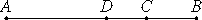# Proposition 45

A major straight line is divided at one point only.

Let AB be a major straight line divided at C, so that AC and CB are incommensurable in square, and let the sum of the squares on AC and CB be rational, but the rectangle AC by CB medial.

I say that AB is not so divided at another point.If possible, let it also be divided at D, so that AD and DB are incommensurable in square and the sum of the squares on AD and DB is rational, but the rectangle contained by them medial.

X.26

Then, since that by which the sum of the squares on AC and CB differs from the sum of the squares on AD and DB is also that by which twice the rectangle AD by DB differs from twice the rectangle AC by CB, while the sum of the squares on AC and CB exceeds the sum of the squares on AD and DB by a rational area, for both are rational, therefore twice the rectangle AD by DB also exceeds twice the rectangle AC by CB by a rational area, though they are medial, which is impossible.

Therefore a major straight line is not divided at different points. Therefore it is only divided at one and the same point.

Therefore, a major straight line is divided at one point only.

Q.E.D.

## Guide

This proposition is not used in the rest of the Elements.